Meijer transform

(diff) ← Older revision | Latest revision (diff) | Newer revision → (diff)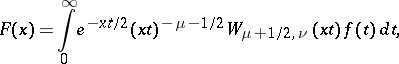where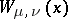is the Whittaker function (cf. Whittaker functions). The corresponding inversion formula is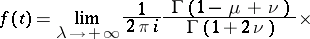For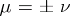the Meijer transform becomes the Laplace transform; for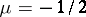it becomes the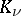-transform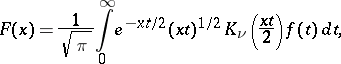where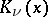is the Macdonald function.

The Varma transform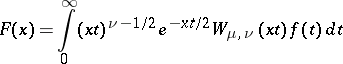reduces to a Meijer transform.

The Meijer-transform (or the Meijer–Bessel transform) is the integral transform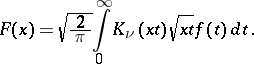If the functionis locally integrable on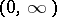, has bounded variation in a neighbourhood of the point, and if the integral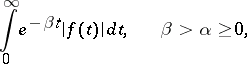converges, then the following inversion formula is valid: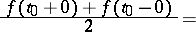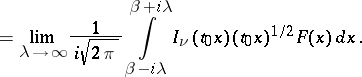For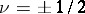the Meijer-transform turns into the Laplace transform.

The Meijer transform and Meijer-transform were introduced by C.S. Meijer in  and, respectively, .